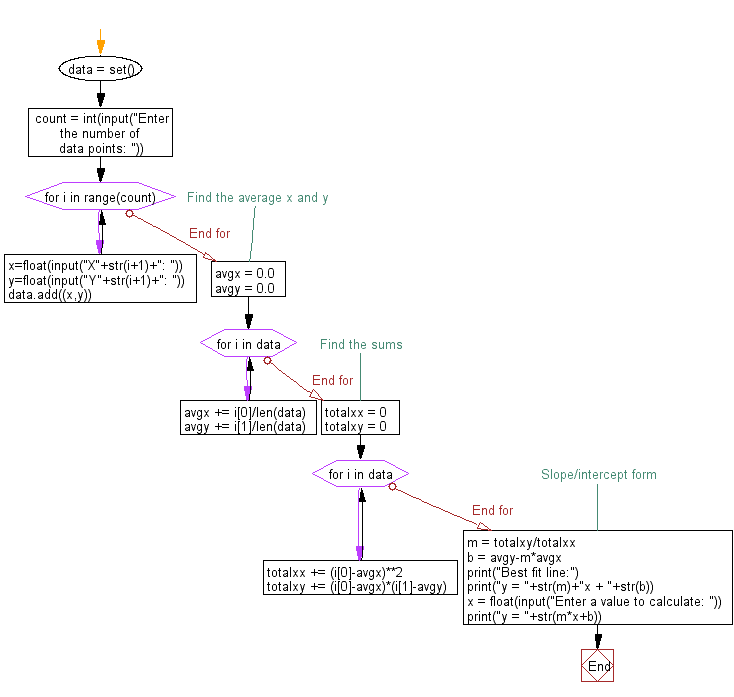﻿ Python Math: Describe linear regression - w3resource# Python Math: Describe linear regression

## Python Math: Exercise-61 with Solution

Write a Python program to describe linear regression .

Note : A linear regression line has an equation of the form Y = a + bX, where X is the explanatory variable and Y is the dependent variable. The slope of the line is b, and a is the intercept (the value of y when x = 0).

Sample Solution:-

Python Code:

``````#https://gist.github.com/cartr/6513044
# Define the data
data = set()
count = int(input("Enter the number of data points: "))
for i in range(count):
x=float(input("X"+str(i+1)+": "))
y=float(input("Y"+str(i+1)+": "))

# Find the average x and y
avgx = 0.0
avgy = 0.0
for i in data:
avgx += i/len(data)
avgy += i/len(data)

# Find the sums
totalxx = 0
totalxy = 0

for i in data:
totalxx += (i-avgx)**2
totalxy += (i-avgx)*(i-avgy)

# Slope/intercept form
m = totalxy/totalxx
b = avgy-m*avgx

print("Best fit line:")
print("y = "+str(m)+"x + "+str(b))

x = float(input("Enter a value to calculate: "))
print("y = "+str(m*x+b))
```
```

Sample Output:

```Enter the number of data points: 2
X1: 1
Y1: 2
X2: 3
Y2: 4
Best fit line:
y = 1.0x + 1.0
Enter a value to calculate: 10
y = 11.0
```

Flowchart:## Visualize Python code execution:

The following tool visualize what the computer is doing step-by-step as it executes the said program:

Python Code Editor:

Have another way to solve this solution? Contribute your code (and comments) through Disqus.

What is the difficulty level of this exercise?

Test your Programming skills with w3resource's quiz.

﻿

```>>> students = [{'name': 'John', 'score': 98}, {'name': 'Mike', 'score': 94}, {'name': 'Jennifer', 'score': 99}]